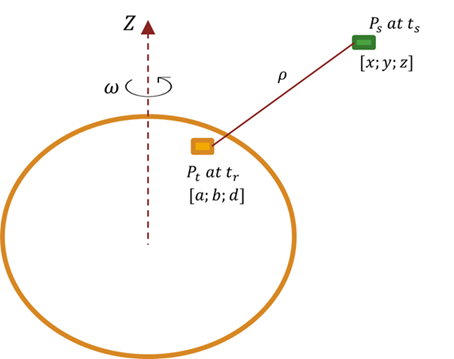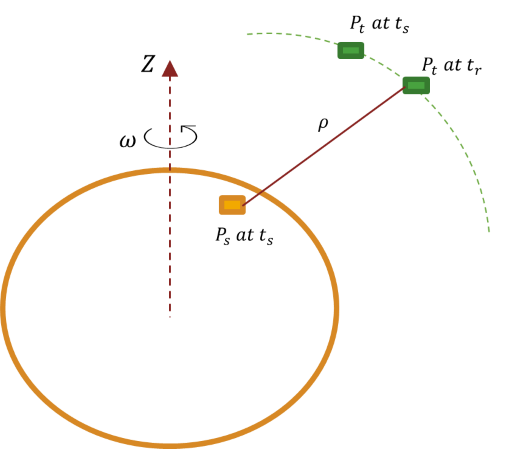# latency

Calculate propagation delay from one asset to another asset

Since R2023a

## Syntax

``delay = latency(source,target)``
``[delay,timeOut] = latency(source,target)``
``[___] = latency(source,target,timeIn)``

## Description

````delay = latency(source,target)` calculates the history of propagation delays from the `source` asset to the `target` asset. The `source` and `target` assets must belong to the same `satelliteScenario` object. If the value of the `AutoSimulate` property of the `satelliteScenario` object is `true`, the `latency` function returns the propagation delay history from the value of `StartTime` to the value of `StopTime`. Otherwise, it returns the propagation delay history from `StartTime` to `SimulationTime`.```
````[delay,timeOut] = latency(source,target)` returns the history of time samples `timeOut`. ```
````[___] = latency(source,target,timeIn)` calculates latency at the specified datetime `timeIn`. When using this syntax, the `latency` function sets the second dimension of `delay` and `timeOut` to `1`. NoteWhen the `target` asset is a `Satellite` object, the `latency` function uses a numerical iterative solution to compute the propagation delay. When the `target` asset is a `GroundStation` object, the `latency` function applies Sagnac correction to compute the propagation delay. ```

## Examples

collapse all

Determine the history of propagation delay from a satellite to a ground station. Also, determine the corresponding time samples.

Create a `satelliteScenario` object.

```startTime = datetime(2022,8,21); % August 21, 2022, 12:00 AM UTC stopTime = datetime(2022,8,23); % August 23, 2022, 12:00 AM UTC sampleTime = 60; % Units are in seconds sc = satelliteScenario(startTime,stopTime,sampleTime);```

Add a satellite to the scenario.

```semiMajorAxis = 10000000; % In meters eccentricity = 0; inclination = 0; % In degrees rightAscensionOfAscendingNode = 0; % In degrees argumentOfPeriapsis = 0; % In degrees trueAnomaly = 0; % In degrees sat = satellite(sc,semiMajorAxis,eccentricity,inclination,rightAscensionOfAscendingNode,argumentOfPeriapsis,trueAnomaly);```

Add a ground station to the scenario.

`gs = groundStation(sc);`

Determine the propagation delay history between two assets and the corresponding time samples.

`[delay,timeOut] = latency(sat,gs);`

## Input Arguments

collapse all

Source asset from scenario, specified as a `Satellite` object, `GroundStation` object, array of `Satellite` objects, or array of `GroundStation` objects.

Target asset from scenario, specified as a `Satellite` object, `GroundStation` object, array of `Satellite` objects, or array of `GroundStation` objects.

Time instance at which the function calculates latency, specified as a datetime scalar. If `timeIn` does not specify a time zone, the function uses Coordinated Universal Time (UTC).

Data Types: `datetime`

## Output Arguments

collapse all

Delay, in seconds, returned as a scalar, vector, or matrix. When `source` and `target` cannot access each other, the corresponding latency value is `NaN`. These tables show how this output changes based on the different input argument sizes and combinations.

• `timeIn` not specified

`source``target``delay`Description
scalarscalarrow vectorLatency history, in which each element represents the propagation delay from `source` to `target` at a specific time.
scalarvectormatrixEach row represents the propagation delay from `source` to `target`. The row index indicates the position of the asset in `target`.
vectorscalarmatrixEach row represents the propagation delay from `source` to `target`. The row index indicates the position of the asset in `source`.
vectorvectormatrixEach row represents the propagation delay from `source` to `target`. The row index indicates the position of the assets in `source` and `target`.

• `timeIn` specified

`source``target``delay`Description
scalarscalarscalarLatency at the specified datetime `timeIn`.
scalarvectorcolumn vectorEach element represents the propagation delay from `source` to the corresponding asset in `target` at the specified datetime `timeIn`.
vectorscalarcolumn vectorEach element represents the propagation delay from the corresponding asset in `source` to `target` at the specified datetime `timeIn`.
vectorvectorcolumn vectorEach element represents the propagation delay between the corresponding pair of `source` and `target` assets at the specified datetime `timeIn`.

Data Types: `double`

Time samples between the start and stop time of the scenario, returned as a scalar or vector.

If `timeIn` does not specify a time zone and the `AutoSimulate` property the `satelliteScenario` object is `true`, the `latency` function returns the time sample history from `StartTime` to `StopTime`. Otherwise, it returns the time sample history from the value of `StartTime` to the value of `SimulationTime`.

Data Types: `datetime`

collapse all

### Latency When Ground Station Is Target

Consider a satellite scenario in which a satellite is the source and a ground station on Earth is the target. Because the satellite scenario currently supports only static ground stations, assume that the velocity of the ground station is the same as the angular velocity of Earth.

Given the instant at which the source transmits the signal ${t}_{\text{s}}$ and the instant at which the target receives the signal ${t}_{\text{r}}$, the latency or propagation delay is the difference ${t}_{\text{r}}-{t}_{\text{s}}$. Assume that at every instant, the source transmits the signal. The unknown is the instant at which the signal reaches the target.Consider that the positions of the source and the target are in International Terrestrial Reference Frame (ITRF).

• Source position Ps at ts

• Target position Pt at tr${\left[a,b,d\right]}^{\prime }$

The distance between the target position Pt and the source position Ps in the tr time frame is the distance travelled by the signal $\rho$, where $\rho =‖{P}_{\text{t}}^{{\text{t}}_{\text{r}}}-{P}_{\text{s}}^{{\text{t}}_{\text{r}}}‖.$

To obtain the source position in the tr time frame, apply this rotation matrix to the source position.

`${R}_{{\text{t}}_{\text{s}}}^{{\text{t}}_{\text{r}}}=\left[\begin{array}{ccc}\mathrm{cos}\left(\omega \left({t}_{\text{r}}-{t}_{\text{s}}\right)\right)& \mathrm{sin}\left(\omega \left({t}_{\text{r}}-{t}_{\text{s}}\right)\right)& 0\\ -\mathrm{sin}\left(\omega \left({t}_{\text{r}}-{t}_{\text{s}}\right)\right)& \mathrm{cos}\left(\omega \left({t}_{\text{r}}-{t}_{\text{s}}\right)\right)& 0\\ 0& 0& 1\end{array}\right]$`

In this equation, the time difference ${t}_{\text{r}}-{t}_{\text{s}}$ equals the time taken by the signal to cover the distance $\rho$.

`$\therefore {t}_{\text{r}}-{t}_{\text{s}}=\frac{\rho }{c}.$`

Substitute this value in the rotation matrix to obtain

`${R}_{{\text{t}}_{\text{s}}}^{{\text{t}}_{\text{r}}}=\left[\begin{array}{ccc}\mathrm{cos}\left(\omega \frac{\rho }{c}\right)& \mathrm{sin}\left(\omega \frac{\rho }{c}\right)& 0\\ -\mathrm{sin}\left(\omega \frac{\rho }{c}\right)& \mathrm{cos}\left(\omega \frac{\rho }{c}\right)& 0\\ 0& 0& 1\end{array}\right].$`

The position of source in the time frame tr is

`${P}_{\text{s}}^{{\text{t}}_{\text{r}}}={R}_{{\text{t}}_{\text{s}}}^{{\text{t}}_{\text{r}}}{P}_{\text{s}}^{{\text{t}}_{\text{s}}}.$`

`$\therefore {P}_{\text{s}}^{{\text{t}}_{\text{r}}}=\left[\begin{array}{ccc}\mathrm{cos}\left(\omega \frac{\rho }{c}\right)& \mathrm{sin}\left(\omega \frac{\rho }{c}\right)& 0\\ -\mathrm{sin}\left(\omega \frac{\rho }{c}\right)& \mathrm{cos}\left(\omega \frac{\rho }{c}\right)& 0\\ 0& 0& 1\end{array}\right]\ast \left[\begin{array}{c}x\\ y\\ z\end{array}\right].$`

In this equation,

• $\omega$ is to the order of `1e-5`.

• $\rho$ is to the order of `1e7`.

• c is to the order of `1e-8`.

• $\omega \frac{\rho }{c}$ is to the order of `1e-5`.

As $\omega \frac{\rho }{c}$ is very small, apply small angle approximation ($\mathrm{sin}\left(\theta \right)\approx \theta$ and $\mathrm{cos}\left(\theta \right)\approx 1$) to obtain

`${P}_{\text{s}}^{{\text{t}}_{\text{r}}}=\left[\begin{array}{ccc}1& \omega \frac{\rho }{c}& 0\\ -\omega \frac{\rho }{c}& 1& 0\\ 0& 0& 1\end{array}\right]\ast \left[\begin{array}{c}x\\ y\\ z\end{array}\right].$`

Substitute this value in $\rho =‖{P}_{\text{t}}^{{\text{t}}_{\text{r}}}-{P}_{\text{s}}^{{\text{t}}_{\text{r}}}‖$ to obtain

`$\rho =\sqrt{{\left(\left(x-a\right)+y\omega \frac{\rho }{c}\right)}^{2}+{\left(-x\omega \frac{\rho }{c}+\left(y-b\right)\right)}^{2}+{\left(z-d\right)}^{2}}.$`

Square both sides of this equation to obtain

`${\rho }^{2}=\left({\left(x-a\right)}^{2}+{\left(y\omega \frac{\rho }{c}\right)}^{2}+2\left(x-a\right)\left(y\omega \frac{\rho }{c}\right)\right)+\left({\left(y-b\right)}^{2}+{\left(x\omega \frac{\rho }{c}\right)}^{2}-2\left(y-b\right)\left(x\omega \frac{\rho }{c}\right)\right)+{\left(z-d\right)}^{2}.$`

Rearrange the terms and simplify the equation to obtain

`${\rho }^{2}={\left(x-a\right)}^{2}+{\left(y-b\right)}^{2}+{\left(z-d\right)}^{2}+\left({\left(\omega \frac{\rho }{c}\right)}^{2}\left({x}^{2}+{y}^{2}\right)+2\omega \frac{\rho }{c}\left(bx-ay\right)\right).$`

The true geometric range for the position of source and target in the tr time frame is

`${r}^{2}=\left({\left(a-x\right)}^{2}+{\left(b-y\right)}^{2}+{\left(d-z\right)}^{2}\right).$`

Let ${R}_{\text{corr}}=\frac{\omega }{c}\left(bx-ay\right)$ and ${e}_{1}{}^{\prime }={\left(\frac{\omega }{c}\right)}^{2}\left({x}^{2}+{y}^{2}\right)$. Substitute these values in ${\rho }^{2}={\left(x-a\right)}^{2}+{\left(y-b\right)}^{2}+{\left(z-d\right)}^{2}+\left({\left(\omega \frac{\rho }{c}\right)}^{2}\left({x}^{2}+{y}^{2}\right)+2\omega \frac{\rho }{c}\left(bx-ay\right)\right)$ to obtain

`${\rho }^{2}={r}^{2}+\left({e}_{1}{}^{\prime }{\rho }^{2}+2\rho {R}_{\text{corr}}\right).$`

`$\therefore \left(1-{e}_{1}{}^{\prime }\right){\rho }^{2}-2\rho {R}_{\text{corr}}-{r}^{2}=0.$`

The discriminant of this quadratic equation is

`$discriminant=4{R}_{\text{corr}}^{2}+4\left(1-{e}_{1}{}^{\prime }\right){r}^{2}.$`

`$\therefore \rho =\frac{2{R}_{\text{corr}}+\sqrt{discriminant}}{2\left(1-{e}_{1}{}^{\prime }\right)}.$`

If ${R}_{\text{corr}}\ll r\sqrt{\left(1-{e}_{1}{}^{\prime }\right)}$, then you can express $\rho$ as

`$\rho =\frac{2{R}_{\text{corr}}+2r\sqrt{1-{e}_{1}{}^{\prime }}}{2\left(1-{e}_{1}{}^{\prime }\right)}=\frac{{R}_{\text{corr}}}{\left(1-{e}_{1}{}^{\prime }\right)}+\frac{r}{\sqrt{1-{e}_{1}{}^{\prime }}}.$`

### Latency When Satellite Is Target

When the target is a satellite, you must consider the movement of a satellite to know when the satellite receives the signal.

Let f be the function that defines the orbital motion of the satellite, which is nonlinear with no sharp peaks. Also, let PS be the position of the source in Geocentric Celestial Reference Frame (GCRF). The time taken by the signal to reach the target equals the time the target takes to move from its position at the transmission time to its position at the reception time.

You can find the solution for the objective function iteratively by using the Newton-Raphson method.

$ObjectiveFunction\left(t\right)=\left(\frac{‖f\left({t}_{\text{S}}+t\right)-{P}_{\text{S}}‖}{c}\right)-t$, where tS is the source transmit time.

Assuming the satellite does not move, the initial guess to the function is the time the signal takes to reach the satellite.

The root of the objective function is latency. Obtaining the exact root is not always possible because of the numerical precision errors. Therefore, consider the value for which the objective function returns a value less than `2.2204e-16` as the root.This methodology is applicable for any moving target, regardless of source movement.

## Version History

Introduced in R2023a